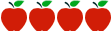### Grade 7: Expressions and Equations

Rating:http://hcpssfamilymath.weebly.com/3-expressions--equations-7ee.html

#### Common Core Standards

Content Standards

7.EE.1 - Apply properties of operations as strategies to add, subtract, factor, and expand linear expressions with rational coefficients.

7.EE.2 - Understand that rewriting an expression in different forms in a problem context can shed light on the problem and how the quantities in it are related.

7.EE.3 - Solve multi-step real-life and mathematical problems posed with positive and negative rational numbers in any form (whole numbers, fractions, and decimals), using tools strategically. Apply properties of operations to calculate with numbers in any form; convert between forms as appropriate; and assess the reasonableness of answers using mental computation and estimation strategies.

7.EE.4a - Solve word problems leading to equations of the form px +q = r and p(x + q) = r, where pq, and r are specific rational numbers. Solve equations of these forms fluently. Compare an algebraic solution to an arithmetic solution, identifying the sequence of the operations used in each approach.

7.EE.4b - Solve word problems leading to inequalities of the form pxq > r or px + q < r, where pq, and r are specific rational numbers. Graph the solution set of the inequality and interpret it in the context of the problem.

7.G.5 - Use facts about supplementary, complementary, vertical, and adjacent angles in a multi-step problem to write and solve simple equations for an unknown angle in a figure.

Standards for Mathematical Practice

MP.1.     Make sense of problems and persevere in solving them.

MP.2.     Reason abstractly and quantitatively.

MP.3.     Construct viable arguments and critique the reasoning of others.

MP.4.     Model with mathematics.

MP.5.     Use appropriate tools.

MP.6.     Attend to precision.

MP.7.     Look for and make use of structure.

MP.8.     Look for and express regularity in repeated reasoning.

#### Description of Unit

In this unit, “Expressions and Equations” from the Howard County Public School System students will create expressions and equations in one variable and use these equations to solve problems.  Students will use properties of operations to generate equivalent expressions. Students explore and solve real-life mathematical problems using numerical and algebraic expressions and equations. In preparation for work on congruence and similarity in Grade 8, they gain familiarity with the relationships between angles formed by intersecting lines and solve for unknowns in a figure. The unit includes a variety of tasks that allow students to explore the ways to solve equations and inequalities, while also applying these skills to the geometry domain and real-world contexts. This is one of five units provided by Howard County School System. Although Howard County is in a PARCC state, the assessment criteria still aligns with SBAC.

#### Cautions

• Limit supports for students working below grade level, diverse learners, and those students who are identified as students with disabilities and for English language learners (ELLs).
• While there is formative assessment included in each lesson, teachers will have to provide explicit summative assessments.

#### Rationale for Selection

• Unit provides lessons that support the rigor of the Common Core State Standards for Mathematics.
• Unit provides explicit, detailed teacher resources and materials, as well as additional instructional support. It includes facilitator’s instructions as well as possible student solutions and student work.
• Provides opportunities for students to apply mathematics in real-world situations, as well as makes connections to transfer knowledge across domains.